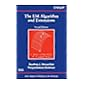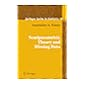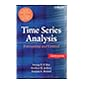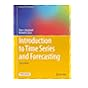Dr. S. R. Lasker Library Online Catalogue

Normal view

# Generalized estimating equations / Andreas Ziegler.

Material type:TextLanguage: English Publication details: 2011. Description: xv, 144 p. : ill. ; 24 cmISBN: 9781461404989 (pbk. : acidfree paper); 1461404983 (pbk. : acidfree paper)Subject(s): Generalized estimating equationsDDC classification: 519.544 LOC classification: QA278.2 | .Z52 2011Online resources: E-book fulltext
Contents:
TOC The linear exponential family -- The quadratic exponential family -- Generalized linear models -- Maximum likelihood method -- Quasi maximum likelihood method -- Pseudo maximum likelihood method based on the linear exponential family -- Quasi generalized pseudo maximum likelihood method based on the linear exponential family -- Algorithms for solving the generalized estimating equations and the relation to the jack-knife estimator of variance -- Pseudo maximum likelihood estimation based on the quadratic exponential family -- Generalized method of moment estimation.
Summary: Summary: Generalized estimating equations have become increasingly popular in biometrical, econometrical, and psychometrical applications because they overcome the classical assumptions of statistics, i.e. independence and normality, which are too restrictive for many problems.Therefore, the main goal of this book is to give a systematic presentation of the original generalized estimating equations (GEE) and some of its Read more...
Tags from this library: No tags from this library for this title.
Star ratingsAverage rating: 0.0 (0 votes)
Holdings
Item type Current library Collection Call number Copy number Status Date due Barcode Item holdsE-Book
E-book
Non-fiction 519.544 ZIG 2011 (Browse shelf(Opens below)) Not For LoanText
Reserve Section
Non-fiction 519.544 ZIG 2011 (Browse shelf(Opens below)) C-1 Not For Loan 26481
Total holds: 0
##### Browsing Dr. S. R. Lasker Library, EWU shelves, Shelving location: Reserve Section Close shelf browser (Hides shelf browser)519.544 MCE 2008 The EM algorithm and extensions / 519.544 MCE 2008 The EM algorithm and extensions / 519.544 TSS 2006 Semiparametric theory and missing data / 519.544 ZIG 2011 Generalized estimating equations / 519.55 BIT 2011 Time series analysis and forecasting by example / 519.55 BOT 2008 Time series analysis : 519.55 BRI 2016 Introduction to time series and forecasting /

Print version:
Ziegler, Andreas, 1966-
Generalized estimating equations.
New York : Springer, 2011
(DLC) 2011931289

Includes bibliographical references (p. 133-141) and index.

TOC The linear exponential family --
Generalized linear models --
Maximum likelihood method --
Quasi maximum likelihood method --
Pseudo maximum likelihood method based on the linear exponential family --
Quasi generalized pseudo maximum likelihood method based on the linear exponential family --
Algorithms for solving the generalized estimating equations and the relation to the jack-knife estimator of variance --
Pseudo maximum likelihood estimation based on the quadratic exponential family --
Generalized method of moment estimation.

Summary:
Generalized estimating equations have become increasingly popular in biometrical, econometrical, and psychometrical applications because they overcome the classical assumptions of statistics, i.e. independence and normality, which are too restrictive for many problems.Therefore, the main goal of this book is to give a systematic presentation of the original generalized estimating equations (GEE) and some of its Read more...

AS

Saifun Momota

There are no comments on this title.